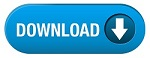Home » SSC » Model Questions for Scientific Assistant (IMD) Exam (Paper-2) PHYSICS – Set 26

# Model Questions for Scientific Assistant (IMD) Exam (Paper-2) PHYSICS – Set 26

a. Infrasonic
b. Ultrasonic
c. Compression
d. Rarefaction

## 2. An input signal is used to change the frequency of a carrier wave. The carrier wave now has regions of higher frequency and regions of lower frequency.

a. Carrier wave
b. Modulation
c. Amplitude Modulation
d. Frequency Modulation

## 3. Two sound waves of slightly different frequencies interfere and cause a throbbing effect.

a. Natural frequency
b. Resonance
c. Beats
d. Carrier wave

a. Resonance
b. Beats
c. Carrier wave
d. Modulation

## 5. An input signal is used to change the amplitude of a carrier wave. The carrier wave now has regions of higher frequency and regions of lower frequency.

a. Carrier wave
b. Modulation
c. Amplitude Modulation
d. Frequency Modulation

### 6. A wave which is used as the base to carry a signal.

a. Beats
b. Carrier wave
c. Modulation
d. Amplitude Modulation

## 7. A force is applied to an elastic object and the force is then removed. The object now oscillates at a certain frequency.

a. Natural frequency
b. Resonance
c. Beats
d. Carrier wave

## 8. A process in which a lower frequency signal wave is impressed onto a higher frequency carrier wave.

a. Carrier wave
b. Modulation
c. Amplitude Modulation
d. Frequency Modulation

## 9. Compressions and rarefactions are not present with

a. longitudinal waves
b. transverse waves
c. both of these
d. neither of these

## 10. All other things being equal, the speed of a sound wave ____ if its wavelength increases.

a. increases
b. decreases
c. both of these
d. neither of these

#